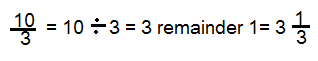# How to Simplify Fractions: Lesson for Kids

Instructor: Bethany Calderwood

Bethany has taught special education in grades PK-5 and has a master's degree in special education.

In this lesson you will learn how to simplify improper and proper fractions. You will find the greatest common factor of the numerator and denominator and use it to reduce the fraction to its lowest terms.

## Making Something Simpler

Ang has a long name - Angela Marianna Cartelloni. She usually only uses her whole name when she wants to sound official. Most people at school call her 'Angela.' Her principal calls her 'Miss Cartelloni' and her music teacher calls her 'Angela Marianna.' All of these names refer to the same person. But her favorite name is her nickname 'Ang.' 'Ang' is the simplest form her name can take while still having the same value or meaning. In the same way that Ang simplifies her name, a mathematician wants to simplify fractions - taking larger numbers and making them smaller numbers with equivalent value.

## Fraction Review

A fraction represents a part of a whole number. It has a denominator on the bottom that tells how many parts are in the whole, and it has a numerator on top that tells how many parts the fraction represents. So the fraction 2/4 represents 2 parts out of a possible 4. When you are working with fractions, it is often necessary to simplify them. When you simplify a fraction, you write it using the smallest numerator and denominator that have the same value as the original fraction.

## Simplifying Improper Fractions

One type of fraction that needs to be simplified is called an improper fraction. An improper fraction has a larger number in the numerator than it has in the denominator. That means that the value of the fraction is greater than one, and it should be turned into a mixed number - a whole number and a fraction.

How do you simplify an improper fraction? Let's find out using the improper fraction 10/3.Remember, the fraction line means ''divided by.'' So 10/3 is 10 divided by 3.

The answer is 3, with a remainder of one. The 3 is the whole number. The remainder becomes the fraction's new numerator, and the fraction's denominator is still 3. The simplified fraction is 3 1/3.

## Simplifying Proper Fractions

Many fractions that are proper fractions (with smaller numerators and larger denominators) are still not in their simplest form. They can be reduced to an equivalent fraction that refers to the same value, just like both the name 'Angela Marianna Cartelloni' and the name 'Ang' refer to the same person.

### Step One: Find the Factors

Let's simplify the fraction 3/12.

The first step is to find the factors of the numerator and of the denominator. The factors of a number are numbers by which it can be divided evenly.

Factors of 3: 1, 3

To unlock this lesson you must be a Study.com Member.

### Register to view this lesson

Are you a student or a teacher?

#### See for yourself why 30 million people use Study.com

##### Become a Study.com member and start learning now.
Back
What teachers are saying about Study.com

### Earning College Credit

Did you know… We have over 200 college courses that prepare you to earn credit by exam that is accepted by over 1,500 colleges and universities. You can test out of the first two years of college and save thousands off your degree. Anyone can earn credit-by-exam regardless of age or education level.